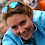## Thursday, September 6, 2012

### Drawing jointly distributed non-normal random variables

* This method only approximates joint non-normal draws (which is really what any method does).

* I was recently told that it was "impossible" to draw joint non-normal distributions.

* But you will see that the approximation looks pretty good.

* It is easy to draw jointly distributed non-normal draws so long as you can start by drawing jointly distributed normal draws.

set more off

clear
set obs 10000

* For instance let's draw four variables.
* 1. a chi2 with 5 degrees of freedom
* 2. a poisson k = 5
* 3. a uniform variable with min = -5 and max = 5
* 4. a random f distribution draw with 5 and 5 degrees for numerator and denominator degrees of freedom.

* First we will specify the correlation matrix.
* The only constraint as far as I know is that the covariance matrix has to be PSD.
* This in practicality limits the possible correlations between variables since cross terms tend to cause vialations more likely in the PSD requirement.

matrix c = (  1, .7,-.3,  .2 \ ///
.7,  1, .2, -.1 \ ///
-.3, .2,  1,  .3 \ ///
.2,-.1, .3,   1 )

* If we do not specify a mean or covariance matrix then the default draws are standard normals which is what we want for simplicity.
drawnorm x1 x2 x3 x4, corr(c)

corr x?
spearman x?

* Now all we need to do is turn our normal draws into uniform draws.
* Note: if x~N(0,1) and THETA is the CDF of the normal then y=CDF(x)~uniform
* So for any new distribution with CDF ALPHA and inverse INVALPHA the variable z=INVALPHA(y) ~ alpha.

gen y1 = normal(x1)
gen y2 = normal(x2)
gen y3 = normal(x3)
gen y4 = normal(x4)

sum
* Looking good.  The next step is that we take the inverse CDF of the distributions of interest.

gen z1 = invchi2(5, y1)
label var z1 "chi2"
* The inverse poisson distribution seems to be incorrectly defined in Stata so that it uses 1-p rather than p to calucalate the inverse.
gen z2 = invpoisson(5, 1-y2)
label var z2 "Poisson"
* It is easy to transform a uniform (0,1) to (a,b) by subtracting a and multiplying by (b-a)
gen z3 = y3*10-5
label var z3 "Uniform"
gen z4 = invF(5, 5, y4)
label var z4 "F distribution"
corr z?
spearman z?
* We can see that the spearman rank correlation is maintained with the standard pearson correlations are only slightly diminished by the non-linear transformations.

* In general the correlations are slightly drawn towards zero so if possible it might be worth it to exagerate the correlations in the matrix c so that they end up being drawn more closely to the desired levels.

hist z1, saving(chi2, replace) nodraw
hist z2, saving(poisson, replace) nodraw
hist z3, saving(normal, replace) nodraw
hist z4, saving(invF, replace) nodraw

graph combine chi2.gph poisson.gph normal.gph invF.gph

* Much of the content of this post was covered in a previous post under the title: Drawing Rank Correlated Random Variables.  It might be worth looking over the previous post if you have additional questions.

1.2.